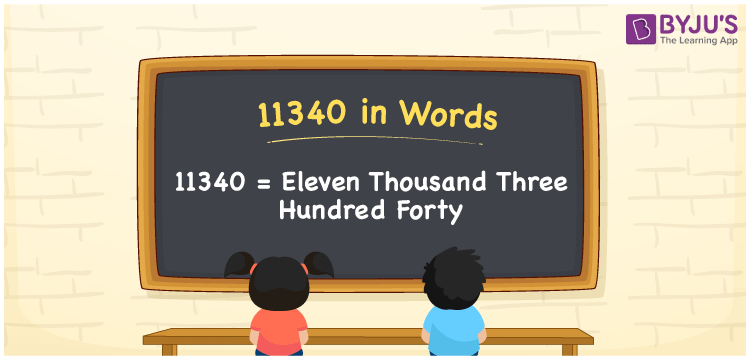# 11340 in Words

11340 in words is Eleven Thousand Three Hundred Forty. For example, if you purchased a camera for Rs. 11340 in a month, you can write, “I have purchased a camera for Rs. Eleven Thousand Three Hundred Forty in a month”. In general, the number name of any number can be written using the ones, tens, hundreds, and thousands place of a number. Thus, the place value chart is useful for writing the number 11340 in words.

 11340 in words Eleven Thousand Three Hundred Forty Eleven Thousand Three Hundred Forty  in Numerals 11340

## 11340 in English Words## How to Write 11340 in Words?

We can convert 11340 to words using a place value chart. This can be done as follows. The number 11340 has 5 digits, so let’s make a chart that shows the place value up to 5 digits.

 Ten thousands Thousands Hundreds Tens Ones 1 1 3 4 0

Thus, we can write the expanded form as:

1 × Ten thousand + 1 × Thousand + 3 × Hundred + 4 × Ten + 0 × One

= 1 × 10000 + 1 × 1000 + 3 × 100 + 4 × 10 + 0 × 1

= 11340

= Eleven Thousand Three Hundred Forty

11340 is the natural number that is succeeded by 11339 and preceded by 11341.

11340 in words – Eleven Thousand Three Hundred Forty

Is 11340 an odd number? – No.

Is 11340 an even number? – Yes

Is 11340 a perfect square number? – No

Is 11340 a perfect cube number? – No

Is 11340 a prime number? – No

Is 11340 a composite number? – Yes

## Frequently Asked Questions on 11340 in Words

Q1

### How to write 11340 in words?

11340 in English can be written as “Eleven Thousand Three Hundred Forty ”.
Q2

### Is the number 11340 an odd number?

No, the number 11340 is not an odd number.
Q3

### Is 11340 a composite number?

Yes, 11340 is a composite number.
Test your Knowledge on 11340 in Words